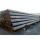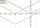# Cinema 4

In cinema are 1656 seats and in the last row are 105 seats , in each next row 3 seats less. How many are the total rows in cinema?

Result

n =  23

#### Solution:

1. a(1) = 105; S(1) = 105
2. a(2) = 102; S(2) = 207
3. a(3) = 99; S(3) = 306
4. a(4) = 96; S(4) = 402
5. a(5) = 93; S(5) = 495
6. a(6) = 90; S(6) = 585
7. a(7) = 87; S(7) = 672
8. a(8) = 84; S(8) = 756
9. a(9) = 81; S(9) = 837
10. a(10) = 78; S(10) = 915
11. a(11) = 75; S(11) = 990
12. a(12) = 72; S(12) = 1062
13. a(13) = 69; S(13) = 1131
14. a(14) = 66; S(14) = 1197
15. a(15) = 63; S(15) = 1260
16. a(16) = 60; S(16) = 1320
17. a(17) = 57; S(17) = 1377
18. a(18) = 54; S(18) = 1431
19. a(19) = 51; S(19) = 1482
20. a(20) = 48; S(20) = 1530
21. a(21) = 45; S(21) = 1575
22. a(22) = 42; S(22) = 1617
23. a(23) = 39; S(23) = 1656Leave us a comment of example and its solution (i.e. if it is still somewhat unclear...):

Showing 0 comments:Be the first to comment!#### To solve this example are needed these knowledge from mathematics:

Looking for help with calculating roots of a quadratic equation? Do you have a linear equation or system of equations and looking for its solution? Or do you have quadratic equation?

## Next similar examples:

1. SeatsSeats in the sport hall are organized so that each subsequent row has five more seats. First has 10 seats. How many seats are: a) in the eighth row b) in the eighteenth row
2. TubesIron tubes in the warehouse are stored in layers so that each tube top layer fit into the gaps of the lower layer. How many layers are needed to deposit 100 tubes if top layer has 9 tubes? How many tubes are in bottom layer of tubes?
3. Equation with abs valueHow many solutions has the equation ? in the real numbers?
4. Solve 3Solve quadratic equation: (6n+1) (4n-1) = 3n2
5. Theorem proveWe want to prove the sentense: If the natural number n is divisible by six, then n is divisible by three. From what assumption we started?
6. Quadratic equationFind the roots of the quadratic equation: 3x2-4x + (-4) = 0.
7. Sequence 3Write the first 5 members of an arithmetic sequence: a4=-35, a11=-105.
8. EquationEquation ? has one root x1 = 8. Determine the coefficient b and the second root x2.
9. SequenceBetween numbers 1 and 53 insert n members of the arithmetic sequence that its sum is 702.
10. AS sequenceIn an arithmetic sequence is given the difference d = -3 and a71 = 455. a) Determine the value of a62 b) Determine the sum of 71 members.
11. DiscriminantDetermine the discriminant of the equation: ?
12. RootsDetermine the quadratic equation absolute coefficient q, that the equation has a real double root and the root x calculate: ?
13. Reciprocal equation 2Solve this equation: x + 5/x - 6 = 4/11
14. Difference AP 4Calculate the difference of the AP if a1 = 0.5, a2 + a3 = -1.1
15. Quadratic inequationIf 5x + x² > 100, then x is not
16. Quadratic equationQuadratic equation ? has roots x1 = 80 and x2 = 78. Calculate the coefficients b and c.
17. VariableFind variable P: PP plus P x P plus P = 160